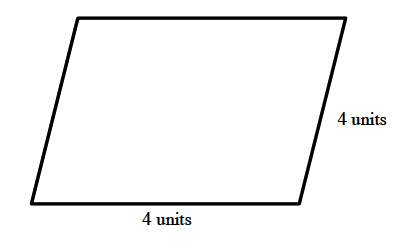### Home > CC1 > Chapter 4 > Lesson 4.2.3 > Problem4-70

4-70.

On graph paper, draw any quadrilateral. Then enlarge (or reduce) it by each of the following ratios.

The quadrilateral below will be used to show you how to do this problem.

1. $\quad \frac { 4 } { 1 }$

To enlarge it by 4:1, each side must be four times as long.
In this case, each side of the parallelogram must be four units long.1. $\quad \frac { 7 } { 2 }$

We can start by making this a ratio in the form of

$\frac{x}{1},$

since our original quadrilateral has sides of length 1 unit. Thus we find the new ratio of

$\frac{7}{2}=\frac{3.5}{1}.$

This means each side of the new quadrilateral must be 3.5 times longer than that of the original.